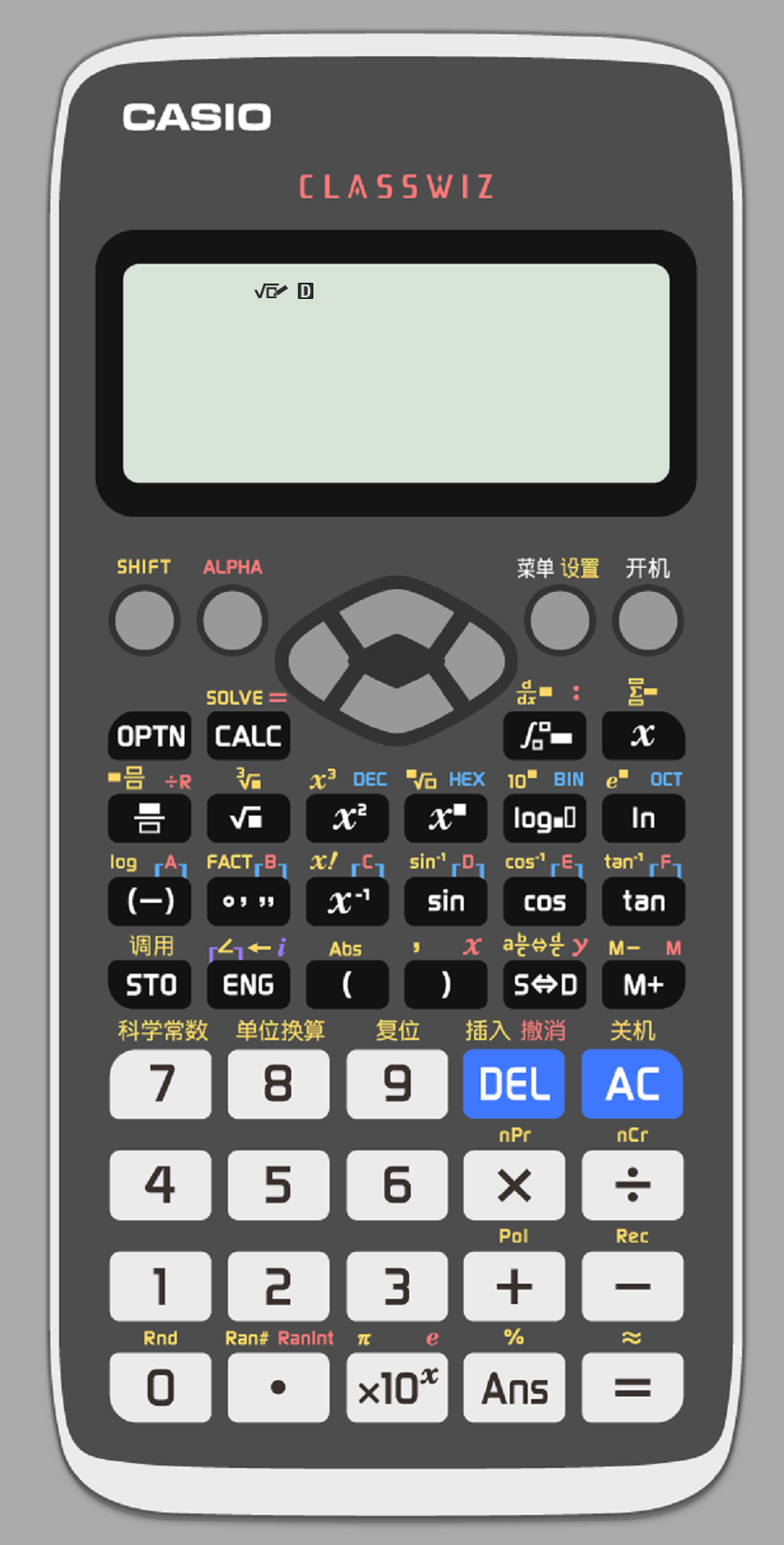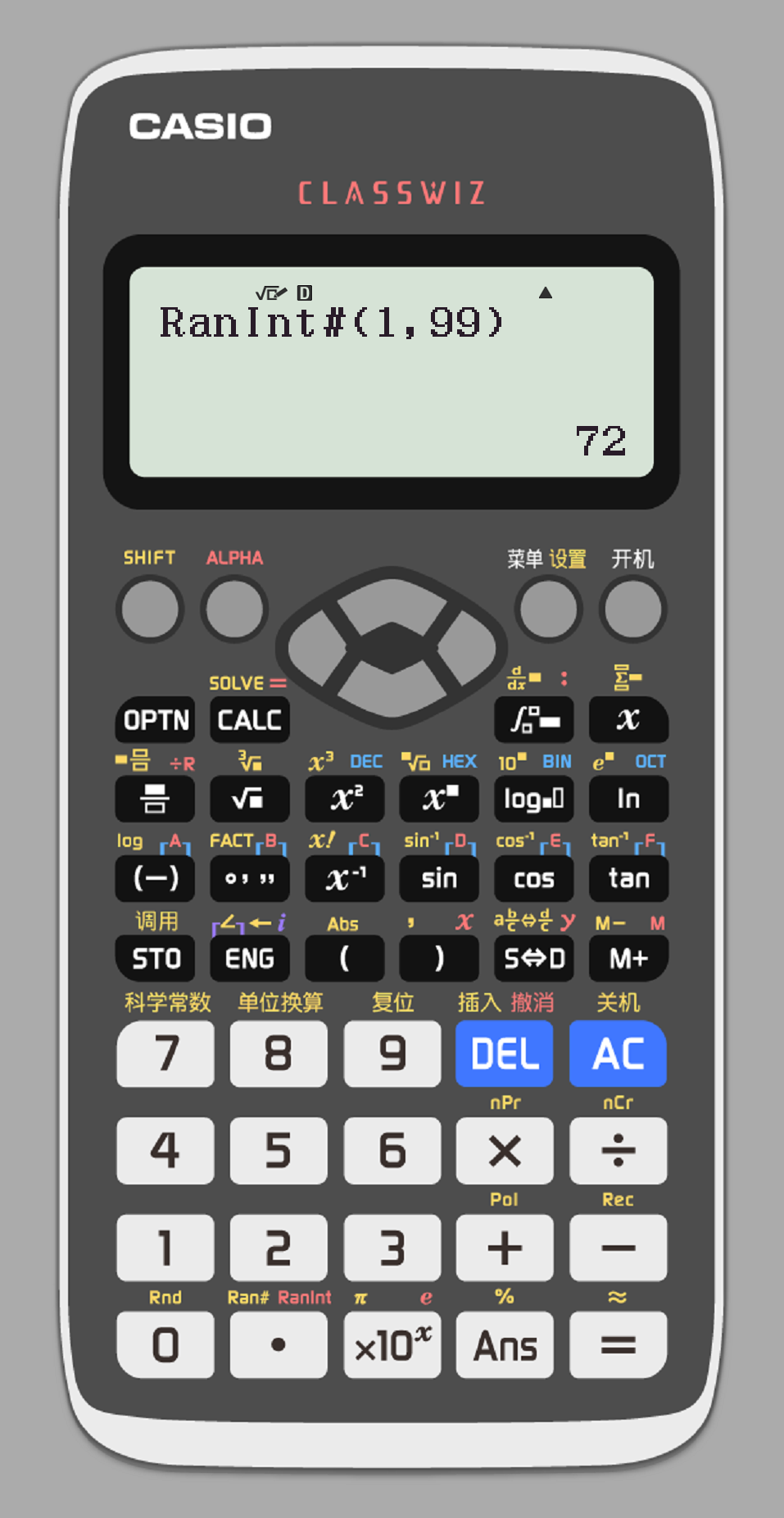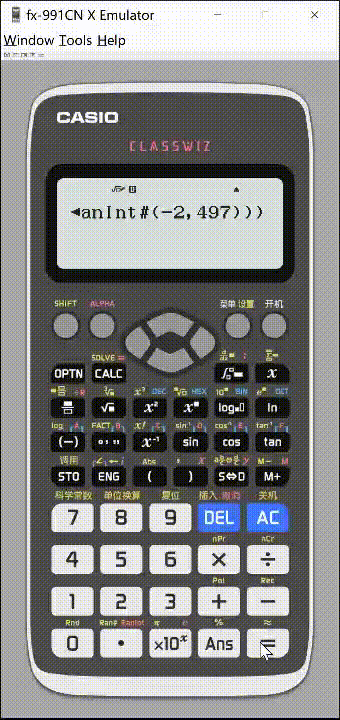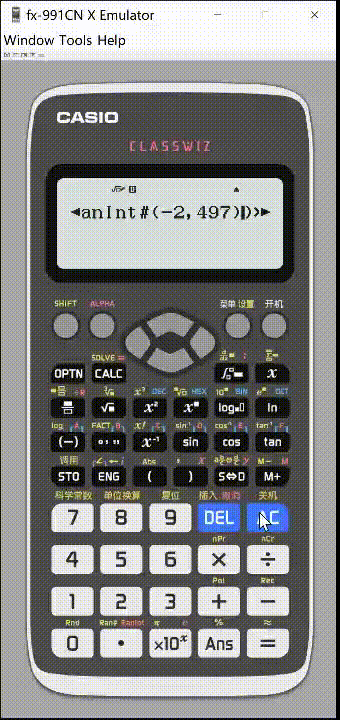## CASIO fx-991CN X学生科学计算器模拟器实现概率发生器（抽卡）CASIO fx-991CN X可谓是广受好评的面向中文用户的科学函数计算器，尽管这不是可写入程序的图形计算器，我们还是可以通过数学表达式来完成一部分功能。

## 原理

Alpha+RanInt+范围可以产生随机整数$$0.6%=0.006=\frac{3}{500}$$

$$\sum_{x=1}^{10}(ln_{RanInt\sharp (-2,497)} )$$## 改进

$$\sum_{x=1}^{10}(ln_{RanInt\sharp (-2,497)}\times 0+1 ) + Ans$$